3.6 Air Columns and Boundary Conditions

Vibrating strings are important to study in the physics of music, as all string instruments are based on properties of the vibrating string. However, there are many other musical instruments that do not involve strings. These are the woodwind and brass instruments. To understand these instruments, we must consider a new type of wave: waves in air. These waves also give rise to sound waves, which are the waves that allows us to hear anything, in the first place!

When discussing waves by using pictures, we must decide how to represent the wave. For the string this was easy. Because we can see the wave, it makes sense to just plot the displacement of the string. But what about a sound wave? We cannot see the wave directly, so its representation is a little more abstract. Sound waves are created when there is a change in pressure. Consider what happens when you make the sound of a "p". The air pressure in your mouth increases until you suddenly release the air making a puffing sound. This disturbance in the air pressure then moves as a wave. So, when we discuss sound waves, we will be talking about the pressure change of the air due to a wave.

Let’s start by considering a narrow tube (like a flute) that is closed at both ends. Initially, the pressure in the tube is constant: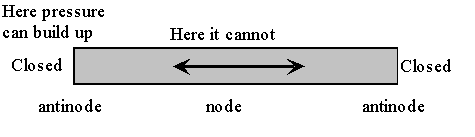The air in the tube can move along the tube. At the ends of the tube the air piles up and can increase the air pressure at that point. In fact, it is at the end of the tube that the pressure increase can be the maximum. So, for a wave in a tube closed at both ends, there will be antinodes at the ends of the tube. Notice that this is just the opposite of vibrating strings. In the middle of the tube, the air moves back and forth but the air pressure does not change – there is nothing for the air to push or pull against to increase or decrease the air pressure. So, there is a node in the middle of the tube (at least for the fundamental mode of vibration). If the air sloshes towards one end of the tube the pressure will increase at that end, but there will now be too few air molecules at the other end of the tube and so the pressure will decrease. However, the high pressure at the one end will then force the air back to the other end, reversing the situation – this is one half of an oscillation of the air in the tube.

Using the fact that the closed tube has antinodes at each end of the tube, we can make plots of the first few modes of vibration: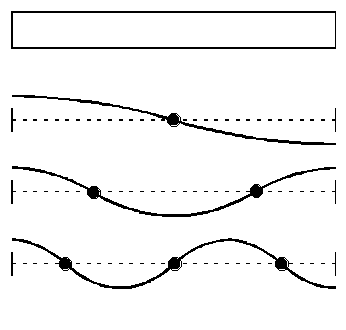If the length of the tube is L, the wavelengths of these modes are: 2L, L, and 2L/3. If we also know the speed of sound, we can determine the frequency of these modes from f = v/l , or v/(2L), v/L, 3v/(2L).

Now, an air column has a new feature compared to strings: we can have a different kind of end, an open end. If a closed end creates a pressure antinode, an open end will have a pressure node: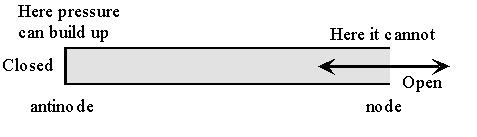What do the lowest modes of vibration look like in this case of one closed end and one open end? Just remember that there must be an antinode at the closed end and a node at the open end: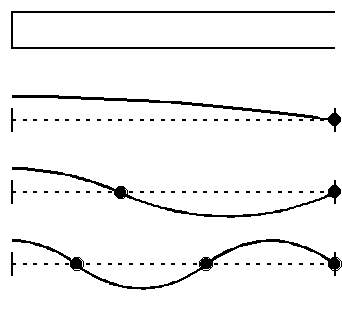Since the distance between a node and antinode is a quarter of a wavelength, l/4, the wavelength of the fundamental mode will be l = 4L. The wavelengths of the next two modes are 4L/3 and 4L/5. The frequencies of these modes will then be v/(4L), 3v/(4L), and 5v/(4L).

In general, whether you have a node or an antinode at one end of a string or tube or any vibrating system is called a boundary condition.

The boundary conditions for an air column are:

Closed end: anti-node.

Open end: node.

These boundary conditions determine the possible modes of vibration. From the modes of vibration, we can determine the wavelength of each mode. Once we have the wavelength, we can find the vibrational frequency from the expression: f× l = v.

As it turns out, different boundary conditions give different overtone series:

A closed-closed tube has a series: 1f, 2f, 3f, …which is a harmonic series.

A closed-open tube has a series: 1f, 3f, 5f, … which is a partial harmonic series.

What about an open-open tube? In this case, there must be a node at each end. If we sketch the possible modes, they will look just like the modes of a vibrating string and will have the same overtone series. So, an open-open tube has a series: 1f, 2f, 3f, …which is a harmonic series.

What effect do these different possibilities have on musical instruments? From the three cases above (c-c, c-o, o-o) we see that there are two possible overtone series: 1f, 2f, 3f, … and 1f, 3f, 5f, … As it turns out, almost all woodwind and brass instruments have a full harmonic series. The one exception is the clarinet; it has the partial harmonics series. This is what gives the clarinet a very distinctive tone – it is more "open" and "pure" because of the fewer harmonics in its tone. But, we will come back to the relationship between tone quality and overtone series, later.

Now, just because the c-c and o-o tube have the same overtone series does not mean that they are identical – their modes of vibration, i.e. the placement of the nodes and antinodes, are quite different and this can affect the placement of finger holes in a wind instrument. Remember that there are two ways to change the pitch of a string: we can press down on a string to shorten the length that vibrates. This raises the pitch of the string. Or, we can lightly touch the string with our finger. This allows the entire string to vibrate, but it imposes a node at the point where we are touching the string. If there is a mode of vibration with a node at that particular point, we will hear that mode, which will have a higher pitch than the fundamental. This technique is used a lot by string players to produce notes with a very high pitch.

The same two ways exist to change the pitch of a wind instrument. First, the length of the tube determines the pitch of the fundamental. Although the length of a flute is always the same, the effective length is determined by how many of the finger holes are covered. The more that are covered, the lower the pitch. However, just as with strings, we can produce higher harmonics on a wind instrument. The effect of an open finger hole is the same as the open boundary condition for a tube: it imposes a node at that point. If there is a vibrational mode with a node at that point, we will hear that particular mode, with it particular pitch.

For example, consider a closed-open tube in its fundamental mode: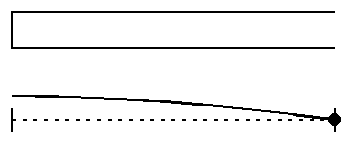What happens now if we open a small hole one third of the way from the closed end of the tube? The air will vibrate if there is a mode of vibration with a node at this same position. As it turns out, there is such a mode: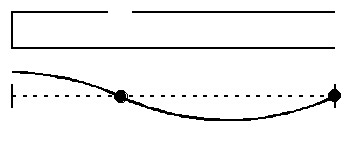In the first case, the mode had a frequency of f, while in the second case, the frequency is raised to 3f. By what interval(s) is the pitch raised by introducing that finger hole?

Vibrating string and air columns form the basis of all melodic instruments in an orchestra and the physics of these two systems determine how these instruments sound and are played. We will discuss the other major class of instruments in the next chapter and explore what makes the fundamental difference between melodic and percussion instruments.

End correction:

Before we leave the topic of air columns, we must deal with one very significant correction to the resonant frequency of an air column – one that has a big effect on the construction of wind instruments. This effect is called the "end correction" and it concerns the open end of a tube. Above, we stated that there is a pressure node at the open end of an air column. This is essentially correct, except that the node is not precisely at the end of the tube; it is a little way outside the tube: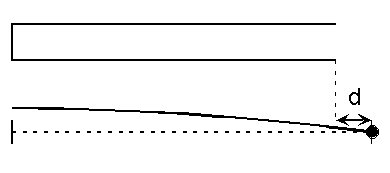The question is how much beyond the end of the tube does the node occur. Again, one needs calculus to figure this out, but scientists have calculated that the extra distance, d, is related to the radius of the tube in the following way:

The end correction, d = 0.6x(radius of the tube).

So, a very thin tube has almost no end correction, while a fat tube will have a large end correction. How does the end correction affect the resonant frequencies of the tube? It is fairly straightforward to take this into account. We generally say that the tube has a length L, in finding the wavelengths of the different modes. However, it is not the length that really matters, it is the distance between nodes and antinodes. The node sits at a position L+d from the other end of the tube. So, we can simply say that the tube has an effective length of L+d. We use the term effective length, because the tube acts as though that is its length, even if its physical length is still L. Notice that is we have an open-open tube, we have to apply the end correction twice: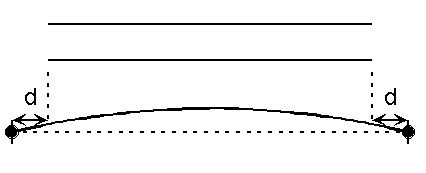For example, take a closed-open tube with a length of 30 cm and a diameter of 4 cm. Without the end correction, the wavelength of the fundamental would be 4L = 120 cm. This would give a fundamental frequency of v/l = 343/1.2 Hz = 286 Hz. Now, the end correction tells us that we should use an effective length of L + 0.6x(radius of tube) = 30 cm + 0.6(2 cm) = 31.2 cm. Remember, the radius of this tube is 2 cm. So now, the wavelength of the fundamental is 4x31.2 cm = 124.8 cm and the frequency of the fundamental is 343/1.248 Hz = 275 Hz. How significant is this? The actual frequency of the tube (275 Hz) is almost a half step lower in pitch than the frequency that we calculated without the end correction. So, musically, this is a big effect.